×#### Thank you for registering.

One of our academic counsellors will contact you within 1 working day.

Click to Chat

1800-1023-196

+91-120-4616500

CART 0

• 0

MY CART (5)

Use Coupon: CART20 and get 20% off on all online Study Material

ITEM
DETAILS
MRP
DISCOUNT
FINAL PRICE
Total Price: Rs.

There are no items in this cart.
Continue Shopping• Complete JEE Main/Advanced Course and Test Series
• OFFERED PRICE: Rs. 15,900
• View Details

```Chapter 15: Linear Inequations – Exercise 15.1

Linear Inequations – Exercise – 15.1 – Q.1

Now, 12x < 50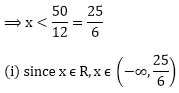(ii) since x ϵ Z, x ϵ{…, -3, -2, -1, 0, 1, 2, 3, 4}

(iii) since x ϵ N, x ϵ {1, 2, 3, 4}

Linear Inequations – Exercise – 15.1 – Q.2

Now,  - 4x > 30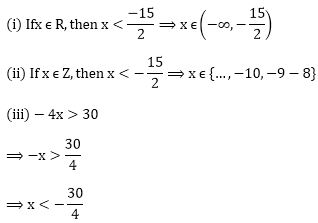As x ϵ N, so x can not be less than 1.

∴ The solution set of the inequality - 4x > 30 is null set Φ.

Linear Inequations – Exercise – 15.1 – Q.3

Now, 4x – 2 < 8

⟹ 4x < 8 + 2

⟹ 4x < 10Linear Inequations – Exercise – 15.1 – Q.4

3x -7 > x + 1

⟹ 3x – x > 1 + 7

⟹ 2 x > 8

⟹ x > 8/2 = 4

⟹ x > 4

∴ (4, ∞) is the solution set.

Linear Inequations – Exercise – 15.1 – Q.5

x + 5 > 4x - 10

⟹ x - 4x > - 10 - 5

⟹ - 3x > - 15

⟹ 3x < 15

⟹ x < 15/3 = 5

⟹ x < 5

∴ (-∞, 5) is the solution set

Linear Inequations – Exercise – 15.1 – Q.6

3x + 9≥ - x + 19

⟹ 3x + x ≥ 19 - 9

⟹ 4x ≥ 10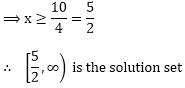Linear Inequations – Exercise – 15.1 – Q.7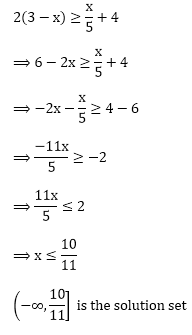Linear Inequations – Exercise – 15.1 – Q.8Linear Inequations – Exercise – 15.1 – Q.9

- (x - 3) + 4 < 5 - 2x

⟹ -x + 3 + 4 < 5 - 2x

⟹ -x + 7 < 5 - 2x

⟹ -x + 2x < 5 - 7

⟹ x < – 2

(- ∞, - 2) is the solution set

Linear Inequations – Exercise – 15.1 – Q.10Linear Inequations – Exercise – 15.1 – Q.11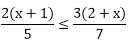⟹ 7(2(x - 1)) ≤ 5(3(2 + x))

⟹ 14(x - 1) ≤ 15(2 + x)

⟹ 14x – 14 ≤ 30 + 15x

⟹ 14x – 15 x ≤ 30 + 14

⟹- x ≤ 44

⟹ x ≥ - 44

∴ The solution set is [- 44, ∞)

Linear Inequations – Exercise – 15.1 – Q.12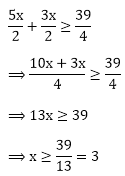⟹ x ≥ 3

∴ The solution set is [3, ∞)

Linear Inequations – Exercise – 15.1 – Q.13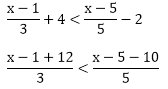5(x – 1 + 12) < 3(x – 5 - 10)

5(x + 11) < 3(x - 15)

5x + 55 < 3x - 45

5x - 3x < - 45 - 55

2x < - 100

x < - 50

∴ The solution set is (-∞, - 50)

Linear Inequations – Exercise – 15.1 – Q.143(2x + 3 - 12) <4 (x – 4 - 6)

3(2x - 9) <4 (x - 10)

6x – 27 < 4x - 40

6x - 4x < - 40 + 27

2x < - 13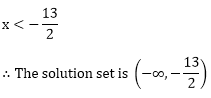Linear Inequations – Exercise – 15.1 – Q.15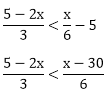6(5 - 2x) <3 (x - 30)

30 – 12 x < 3x - 90

- 12x - 3x < - 90 - 30

- 15x < - 120

15x < - 120

x > 120/15 = 8

∴ The solution set is (8, ∞)

Linear Inequations – Exercise – 15.1 – Q.16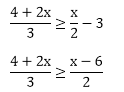2(4 + 2x) ≥ 3(x - 6)

8 + 4 x ≥ 3x - 18

4x - 3x ≥ - 18 - 8

X ≥ - 26

∴ The solution set is [- 26, ∞)

Linear Inequations – Exercise – 15.1 – Q.172x – 7 < 3x - 6

2x - 3x < - 6 + 7

- x < 1

X > - 1

∴ The solution set is (- 1, ∞)

Linear Inequations – Exercise – 15.1 – Q.18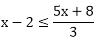3(x - 2) ≤ 5x + 8

3x – 6 ≤ 5x + 8

3x - 5x ≤ 8 + 6

- 2x ≤ 14

2x ≥ - 14

X ≥ - 7

∴ The solution set is [- 7, ∞)

Linear Inequations – Exercise – 15.1 – Q.19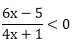Case 1: 6x – 5 > 0 and 4x + 1 < 0This is not possible.

Case 2: 6x – 5 < 0 and 4x + 1 > 0```### Course Features

• 728 Video Lectures
• Revision Notes
• Previous Year Papers
• Mind Map
• Study Planner
• NCERT Solutions
• Discussion Forum
• Test paper with Video Solution